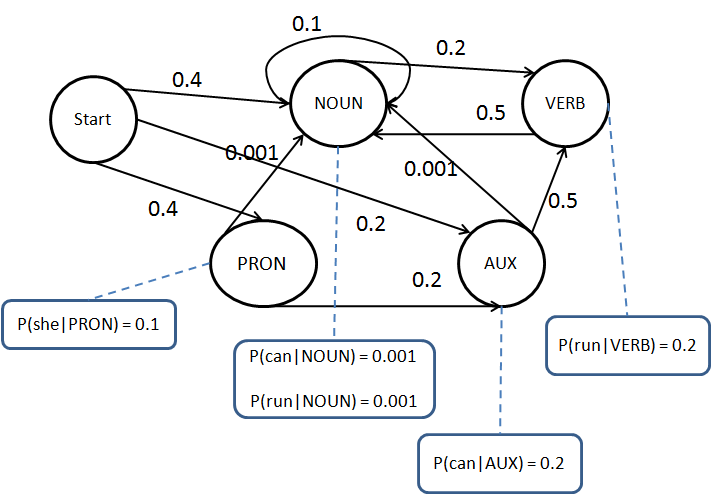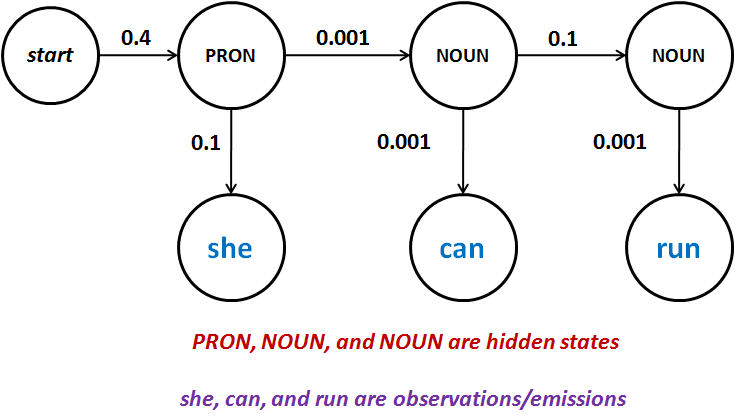Please visit, subscribe and share 10 Minutes Lectures in Computer Science

## POS Tagging using Hidden Markov Model - Solved Exercise

Question:
For the given HMM, show the transition and emission probability matrices. Also, calculate the probability of P(she|PRON can|NOUN run|NOUN).Solution:
As per the HMM, the following are the transition and emission (observation) probabilities;
Transition probabilities
Emission probabilities
 PRON NOUN AUX VERB Start 0.4 0.4 0.2 PRON 0.001 0.2 NOUN 0.1 0.2 AUX 0.001 0.5 VERB 0.5
 she can run PRON 0.1 NOUN 0.001 0.001 AUX 0.2 VERB 0.2
Following graph shows the portion of HMM to solve the given problem;P(she|PRON can|NOUN run|NOUN)
= P(PRON|Start) * P(she|PRON) * P(NOUN|PRON) * P(can|NOUN) * P(NOUN|NOUN) * P(run|NOUN)
= 0.4 * 0.1 * 0.001 * 0.001 * 0.1 * 0.001
= 0.000000000004
= 4*10-12
We arrived at this value by multiplying the transition and emission probabilities.

**********